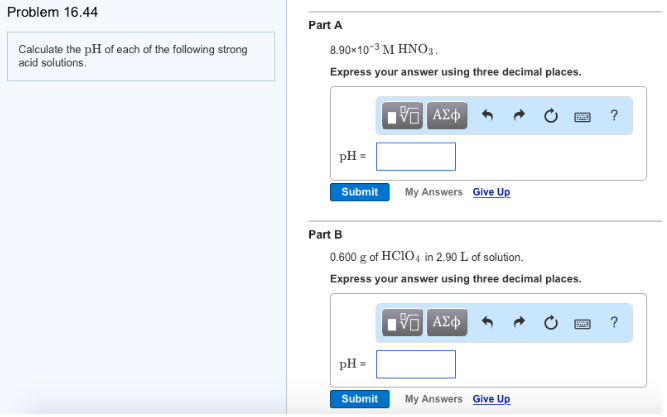# Problem: Calculate the pH of each of the following strong acid solutions. Part A8.90 x 10-3 M HNO3. Express your answer using three decimal places.Part B0.600 g of HClO4 in 2.90 L of solution. Express your answer using three decimal places.

###### FREE Expert Solution
93% (71 ratings)###### Problem Details

Calculate the pH of each of the following strong acid solutions.

Part A

8.90 x 10-3 M HNO3

Part B

0.600 g of HClO4 in 2.90 L of solution.